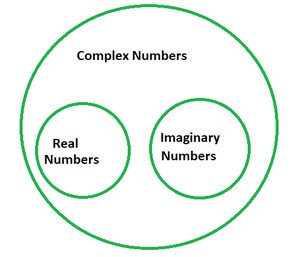Open in App
Not now

# Simplify (-3i)(7i)(-i)

• Last Updated : 09 Mar, 2022

Complex numbers have the form a + ib, in which a and b are real numbers and I (iota) denotes square root of -1, i.e., √-1 and are frequently represented in this form only, also regarded as the rectangular or standard form. For example, 2 + 9i is a complex quantity in which 2 denotes the real component and 9i denotes the imaginary component. They might be entirely genuine or entirely fabricated, based on the value of a and b. So whenever a = 0 in a + ib, ib is a purely fictitious number, while when b = 0, we obtain a, which is strictly real.Iota and its Powers

Iota is a hypothetical unit number denoted by the letter i and its value is √-1, i.e., i = √-1. You may have encountered circumstances when the discriminant is negative while solving quadratic equations. Imagine the equation x2 + x + 1 = 0 as an example. When we solve this problem using the quadratic formula, we get a negative discriminant (the component within the square root). This negative value under the square root is symbolized as iota.

• i = √-1
• i2 = −1
• i3 = i × i2 = i × −1 = −i
• i4 = i2 × i2 = −1 × −1 = 1

Multiplying Imaginary Numbers

We have already established that an imaginary number is of the form 0 + ai. Let us take two imaginary numbers, ai and bi, and see how they would be multiplied. In order to do that, multiply the coefficients of iota separately and the imaginary parts(i) separately, i.e.,

ai × bi = (a × b) × (i × i)

= ab × i2

= ab × -1

ai × bi = -ab

### Simplify (-3i)(7i)(-i)

Solution:

(-3i)(7i)(-i) = (3 × 7) × (-i × i × -i)

= 21 × i3

Since, i3 = -i

⇒ 21 × i3 = 21 × -i

= -21i

### Sample Problems

Question 1. Simplify: (4 + i)(7 + 3i).

Solution:

(4 + i)(7 + 3i) = 4(7 + 3i) + i(7 + 3i)

= 28 + 12i + 7i + 3i2

= 28 + 19i + 3(-1)

= 28 – 3 + 19i

= 25 + 19i

Question 2. Simplify: (2 + i)(-5).

Solution:

(2 + i)(-5) = 2(-5) + i(-5)

= -10 – 5i

Question 3. Simplify: (4 + 8i)(4 – 8i).

Solution:

Using the algebraic identity (a + b)(a – b) = a2 – b2, we have:

(4 + 8i)(4 – 8i) = 42 – (8i)2

= 16 – 82i2

= 16 – 64(-1)

= 16 + 64

(4 + 8i)(4 – 8i) = 80

Question 4. Evaluate: (5 + 6i)(6 – 5i).

Solution:

(5 + 6i)(6 – 5i) = 5(6 – 5i) + 6i(6 – 5i)

= 30 – 25i + 36i – 30i2

= 30 + 11i – 30(-1)

(5 + 6i)(6 – 5i) = 60 + 11i

Question 5. Simplify: (-13i)(17i)(-i).

Solution:

(-3i)(7i)(-i) = (13 × 17) × (-i × i × -i)

= 221 × i3

Since, i3 = -i

⇒ 221 × i3 = 221 × -i

= -221i

My Personal Notes arrow_drop_up
Related Articles